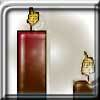#### You may also like### Burning Down

One night two candles were lit. Can you work out how long each candle was originally?### Percentage Unchanged

If the base of a rectangle is increased by 10% and the area is unchanged, by what percentage is the width decreased by ?### Digit Sum

What is the sum of all the digits in all the integers from one to one million?

# Elephants and Geese

##### Age 14 to 16 ShortChallenge Level

Using ratio and currency
Yesterday
1 white elephant and 99 geese both worth $k$

Today
1 white elephant is worth 0.9$k$
99 wild geese are worth 1.1$k$

How many geese can 1 elephant = 0.9$k$ buy?
99 geese worth 1.1$k$
9 geese worth 0.1$k$
81 geese worth 0.9$k$

Using percentage multipliers
Wild geese are now worth 110% of their original price. To match their original value, we can remove the extra 10%.

The white elephant has decreased in price by 10%, so we will need 10% fewer geese to match its price.

Using fractions
10% is $\frac{1}{11}$ of 110%, so removing 10% from 110% is the same as removing $\frac{1}{11}$.
$\frac{1}{11}$ of 99 is 9, so that leaves 90 geese.

Removing 10% of the remaining 90 geese leaves 90$-$9 = 81 geese.

Using decimals
Reducing 110% to 1000% is the inverse of increasing by 10%, so can be done by dividing by 1.1, and reducing by 10% can be done by multiplying by 0.9.

99$\div$1.1$\times$0.9 = 81. So 81 wild geese are worth the same amount as a white elephant.

You can find more short problems, arranged by curriculum topic, in our short problems collection.